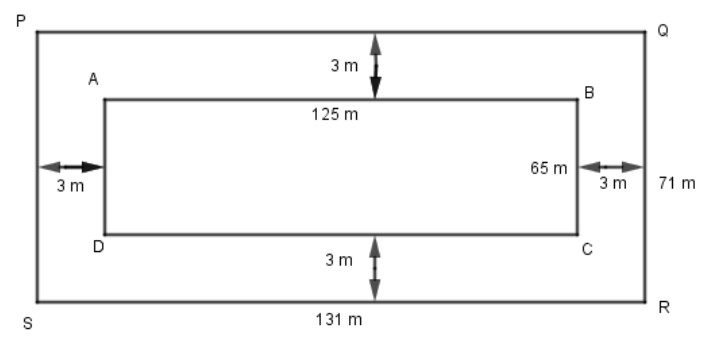QuestionAnswers

# A 3 m wide path runs outside and around a rectangular park of length 125 m and breadth 65 m. Find the area of the path.

Hint: In the above question, to find the area of the path we have to find the area of a park ABCD then we will add the width of path that is 3 m twice to find the length of PQ, in the same way we will find the width of QR by adding 3 m twice. We will find the area of PQRS. Then we will subtract the area of PQRS and the area of ABCD, we will get the area of the path.

Given,
Width of path running outside = 3 m
Length of rectangular park = 125 m
Breadth of rectangular park = 65 mArea of rectangular park ABCD = length X breadth
$= 125 \times 65 = 8,125{m^2}$.
Now, length of PQ = 125 + 3 + 3 = 131 m
Breadth of QR = 65 + 3 + 3 =71 m
$\text{Area of PQRS} = \text{length} \times \text{breadth}$
$= 131 \times 71 = 9,301{m^2}$
Thus, the area of a path = Area of PQRS – Area of ABCD
$= 9,301 - 8,125 = 1176{m^2}$.
Hence, the area of the path is $1176{m^2}$.

So, the correct answer is “Option A”.